# ML Aggarwal Class 7 Solutions for ICSE Maths Chapter 5 Sets Ex 5.1

## ML Aggarwal Class 7 Solutions for ICSE Maths Chapter 5 Sets Ex 5.1

Question 1.
State which of the following collections are set:
(i) All states of India.
(ii) Four cities of India having more than one lac population.
(iii) All tall students of your school.
(iv) Four colours of a rainbow.
(v) All the beautiful flowers.
(vi) All clever people of Lucknow.
(vii) Last three days of a week.
(viii)All months of a year having at least 30 days.
Solution: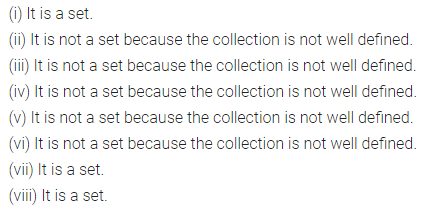Question 2.
Let A = {vowels of English alphabet}, then which of the following statements are true. In case a statement is incorrect, mention why.
(i) c ∈ A
(ii) {a} ∈ A
(iii) a, i, u, ∈ A
(iv) {a, u} ∉ A
(v) {a, i, u } ∈ A
(vi) a, b, ∈ A
Solution:Question 3.
Describe the following sets:
(i) {a, b, c, d, e, f}
(ii) {2, 3, 5, 7, 11, 13, 17, 19}
(iii) {Friday, Saturday, Sunday}
(iv) {April, August, October}
Solution: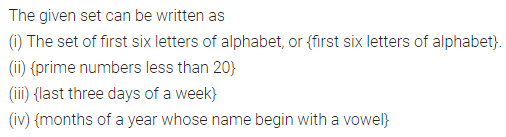Question 4.
Write the following sets in tabular form and also in set builder form:
(i) The set of even whole numbers which lie between 10 and 50.
(ii) {months of a year having more than 30 days}
(iii) The set of single-digit whole numbers which are a perfect square.
(iv) The set of factors of 36.
Solution: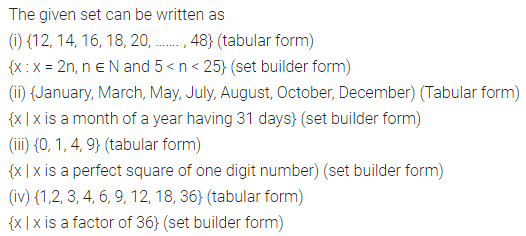Question 5.
Write the following sets in roster form and also in description form:
(i) {x | x = 4n, n ∈ W and n < 5}
(ii) {x : x = n2, n ∈ N and n < 8}
(iii) y : y = 2x – 1, x ∈ W and x < 5}
(iv) {x : x is a letter in word ULTIMATUM}
Solution: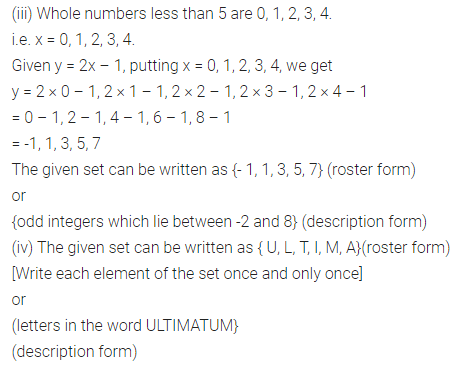Question 6.
Write the following sets in roster form:
(i) {x | x ∈ N, 5 ≤ x < 10 }
(ii) {x | x = 6 p, p ∈ I and – 2 ≤ p ≤ 2}
(iii) {x | x = n2 – 1, n ∈ N and n < 5}
(iv) {x | x – 1 = 0}
(v) {x | x is a consonant in word NOTATION}
(vi) {x | x is a digit in the numeral 11056771}
Solution: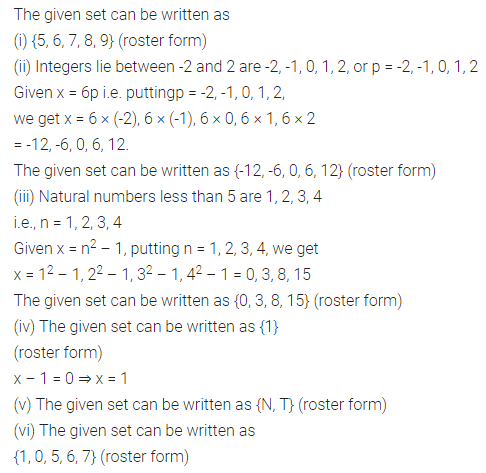Question 7.
Write the following sets in set builder form:
(i) (1, 3, 5, 7, …….. 29}
(ii) {2, 3, 5, 7, 11, 13, 17, 19, 23, 29}
(iii) {1, 4, 9, 16, 25, ………}
(iv) {$$\frac { 1 }{ 5 }$$, $$\frac { 1 }{ 6 }$$, $$\frac { 1 }{ 7 }$$, …… $$\frac { 1 }{ 20 }$$}
(v) {-16, -8, 0, 8, 16, 24, 32, 40}
(vi) {January, June, July}
Solution: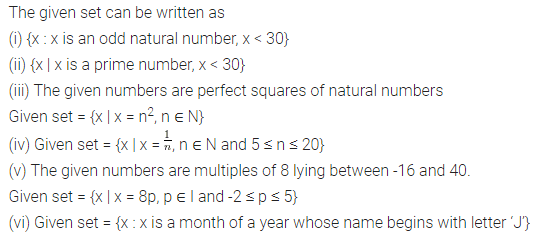Question 8.
If V is the set of vowels in the word COMPETITION, write the given set in
(i) description form
(ii) set builder form
(iii) roster form
Solution: